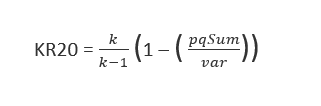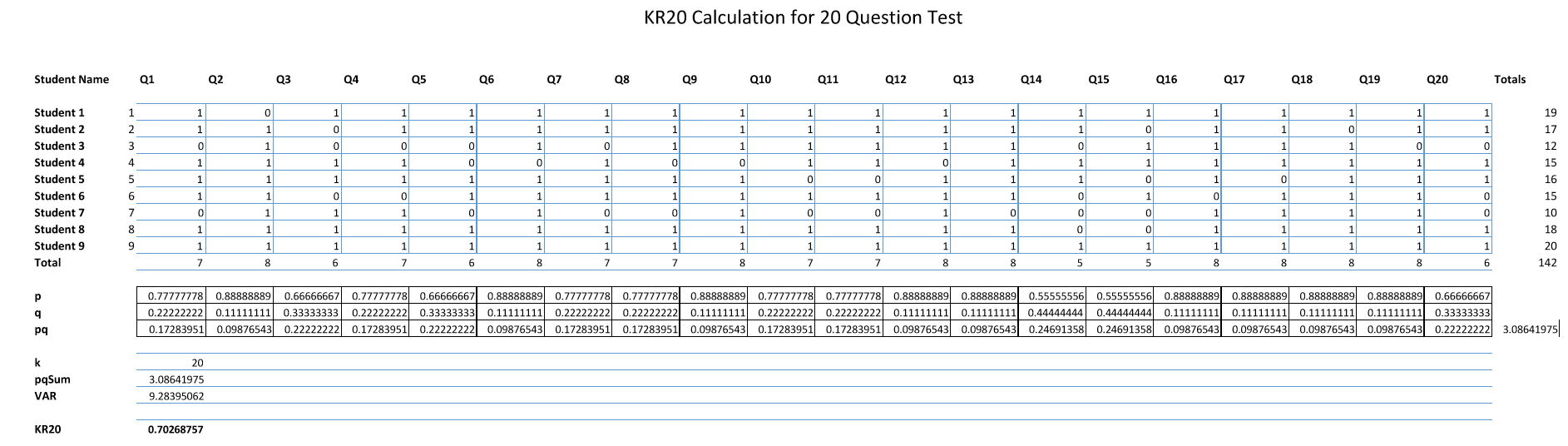# KR20

Sarah Smith -

The Kuder and Richardson Formula 20 (KR20) is used to estimate the reliability of binary measurements, to see if the items within the tests obtained the same binary (right/wrong) results over a population of testing subjects.

The values that appear on your Class Tests Results page should range from 0 to 1.00, although it is mathematically possible to see a negative value reported.  Values that are above .70 are in the acceptable range, while too high a value (above .90) indicates a homogeneous test.

The equation that is used to calculate your test’s KR20 coefficient is:Where:

k = Number of questions on the test

pj = Percentage of students in the class who answered question j correctly

qj = Percentage of students in the class who answered question j incorrectly

pqSum = Total sum of the product of p & q for all questions on the test

var = variance of the total scores of all students that took the test.  (Standard deviation squared)

The KR20 may be affected by the difficulty of the test, the total number of questions on the test, the number of questions on the test that did not discriminate or negatively discriminated, and the spread in the student’s scores. Also, it is important to remember that calculated class values are not accurate until at least 4 students have completed the test.

Here is an example to help illustrate how these scores are calculated:Also please see the attached files (for larger images).